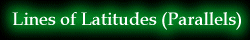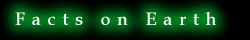page1
Map ProjectionLines of Latitude •Equator •Latitudes Lines of Longitude •Prime Meridian •Longitudes Relationships Facts on Earth Zenithal Projections •Gnomonic Polar •Gnomonic Equatorial •Stereographic Polar •Stereographic Equatorial •Orthographic Polar •Orthographic Equatorial Simple Conic Projection Cylindrical Equal-area Projection ReferencesEquator The equator is the circular line created by the intersection of the spherical earth and a flat plane that is equidistant between the two ends of the polar axis and perpendicular to the axis. From this definition, two conditions can be drawn. Firstly, the center of the circular plane created by the equator is also the center of the earth. Secondly, every point on the equator is equidistant from the two poles. The equator serves many purposes. One of such purposes is to divide the earth into two equal hemispheres - northern and southern. In addition, it serves as a reference point and division for all other lines that are parallel to the equator. Such lines are known as parallels or latitudes. As such, the equator has been numbered 0° with the latitudes divided into those that are north and south the equator. Most importantly, the equator is the largest circle of all the lines of latitudes. Such characteristics make the equator a 'Great Circle.'Latitudes (Parallels) Latitudes or parallels are imaginary lines created by the intersection of a plane with the earth. Such planes are parallel to the equator and are at a constant angular distance from the equator.Thus, latitudes are also perpendicular to the polar axis. Conventionally, the numbering starts from the equator at 0° and increases to both poles at 90°.When drawn at an interval of 1°, there will be 89 circles of latitudes on either side of the equator. Some characteristics of latitudes: The arc between any two parallels a and b will subtend an angle of (a-b) ° at the centre of the globe. There is a complementary arc between the parallel and the pole subtended by an angle of (90-n) ° at the centre. This is called the angle of the co-latitude.Prime Meridian The Prime Meridian is the semi-circular line created by the intersection of the spherical earth and a flat plane. This flat plane lies along the polar axis and goes through the observatory at Greenwich near London, England. In other words, it is a special meridian that bisects the sphere into east and west hemisphere and serves as a reference point for all other meridians. As a result, it has been numbered 0° with the longitude divided into those that are east and west of the Prime Meridian.Longitudes (Meridians) Longitudes or meridians are imaginary lines created by the intersection of a plane with the earth. Unlike latitudes, the edge of such planes touches the polar axis at all points to create a semi-circles (lunes) through the poles. As such, longitudes possess many of the same characteristics as the polar axis. For one, all longitudes would be perpendicular to the equator. Secondly, the centre of the circle created by two opposite meridians would intersect the centre of the earth. Thus, having equal radius to those of the earth and are all equal in size. This also means that they are equal to the equator in size and thus, are also defined to be 'Great Circles.'Conventionally, the numbering starts from the Prime Meridian at 0° and increases east and west of the Prime Meridian to 180°.Therefore, making a complete 360° rotation with 360 equal lunes around the polar axis, which will cover the entire surface of the earth. Some characteristics of longitudes: The arc of the Equator between any two meridians subtends an angle at the centre of the earth equal to the difference between their respective degrees of longitude. Ex. if the meridians are a and b, the angle subtended would be b-a. A similar angle would be made by tangents to these meridians at the Poles. Opposite halves of a meridian are of supplementary degrees of longitude.From the characteristics of parallels and meridians, there comes about several relationships between the two types of lines. Such relationships are listed as follows: Any meridian intersects any parallel at a right angle. With 90 latitudes on either side of the equator, the lines of latitudes will divide the meridian into 180 equal arcs all subtended by 1° at the centre of the sphere and with a length 360th of the circumference. In addition, these arcs of the meridians are equal to the arcs of the equator created by intersections with the meridian. The parallels will be divided into 360 equal arcs by the 180 different meridians on either side of the prime meridian. The length of such arcs decrease from the equator twoards the pole at which point they vanish.In reality, the earth is not a perfect sphereical object due to the constant spinning of the earth on its axis. This motion creates a slight bluge on the surface midway between the two equator. Thus, flattening the surface of the polar regions and distorting the meridians to departure from perfect circles to an extent of 1/297. In addition, the equatorial diameter becomes 26 miles longer than that of the meridian's diameter. The true characteristics possessed by the earth is listed as follows: Equatorial radius: 6, 378.2064 km Equatorial diameter: 12, 756.4128 km Polar semi-axis: 6, 356.5838 km Polar axis: 12, 713.1676 km Equatorial circumference: 40, 057 km Such characteristics has been defined as geoid, which means earth-shaped body.Mainwaring, James. An Introduction to the Study of Map Projection. London: Macmillan and Co. Ltd, 1942. Masood, M. Salar. Map Projections. Mysore: Mysore Printing and Publishing House, 1961. Steers, J.A. An Introduction to the Study of Map Projections. London: University of London Press Ltd, 1962.